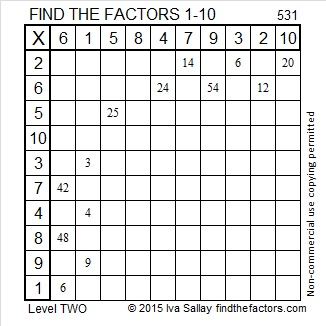# 582 and Level 4

582 is the sum of all the prime numbers from 59 to 89. Can you list those eight consecutive primes?

582 is also the hypotenuse of the Pythagorean triple 390-432-582. Which of 582’s factors is the greatest common factor of those three numbers?Print the puzzles or type the solution on this excel file: 12 Factors 2015-08-10

—————————————————————————————————

• 582 is a composite number.
• Prime factorization: 582 = 2 x 3 x 97
• The exponents in the prime factorization are 1, 1, and 1. Adding one to each and multiplying we get (1 + 1)(1 + 1)(1 + 1) = 2 x 2 x 2 = 8. Therefore 582 has exactly 8 factors.
• Factors of 582: 1, 2, 3, 6, 97, 194, 291, 582
• Factor pairs: 582 = 1 x 582, 2 x 291, 3 x 194, or 6 x 97
• 582 has no square factors that allow its square root to be simplified. √582 ≈ 24.124676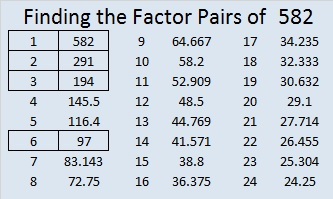—————————————————————————————————# 581 and Level 3

A year ago I wrote a short, but heartfelt post for World Elephant Day. I invite you to read it or any other article that makes you aware of what you can do to protect elephants from poachers.

581 is the sum of consecutive primes two different ways: 191 + 193 + 197 = 581, and the sum of all the prime numbers from 19 to 71 also is 581.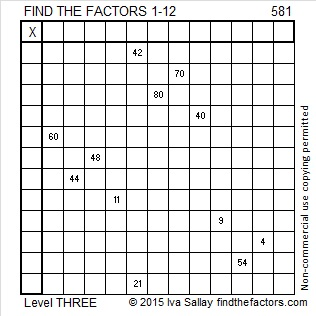Print the puzzles or type the solution on this excel file: 12 Factors 2015-08-10

—————————————————————————————————

• 581 is a composite number.
• Prime factorization: 581 = 7 x 83
• The exponents in the prime factorization are 1 and 1. Adding one to each and multiplying we get (1 + 1)(1 + 1) = 2 x 2 = 4. Therefore 581 has exactly 4 factors.
• Factors of 581: 1, 7, 83, 581
• Factor pairs: 581 = 1 x 581 or 7 x 83
• 581 has no square factors that allow its square root to be simplified. √581 ≈ 24.10394—————————————————————————————————

A Logical Approach to solve a FIND THE FACTORS puzzle: Find the column or row with two clues and find their common factor. Write the corresponding factors in the factor column (1st column) and factor row (top row).  Because this is a level three puzzle, you have now written a factor at the top of the factor column. Continue to work from the top of the factor column to the bottom, finding factors and filling in the factor column and the factor row one cell at a time as you go.# 580 and Level 2

580 is the sum of all the prime numbers from 83 to 107. Do you know what those consecutive prime numbers are?

580 is also the hypotenuse of four Pythagorean triples. In each case can you find the factor of 580 that is the greatest common factor of the triple?

• 68-576-580
• 96-572-580
• 348-464-580
• 400-420-580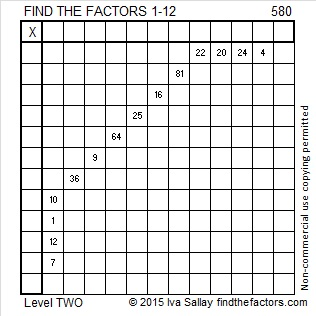Print the puzzles or type the solution on this excel file: 12 Factors 2015-08-10

—————————————————————————————————

• 580 is a composite number.
• Prime factorization: 580 = 2 x 2 x 5 x 29, which can be written 580 = (2^2) x 5 x 29
• The exponents in the prime factorization are 2, 1, and 1. Adding one to each and multiplying we get (2 + 1)(1 + 1)(1 + 1) = 3 x 2 x 2 = 12. Therefore 580 has exactly 12 factors.
• Factors of 580: 1, 2, 4, 5, 10, 20, 29, 58, 116, 145, 290, 580
• Factor pairs: 580 = 1 x 580, 2 x 290, 4 x 145, 5 x 116, 10 x 58, or 20 x 29
• Taking the factor pair with the largest square number factor, we get √580 = (√4)(√145) = 2√145 ≈ 24.083189—————————————————————————————————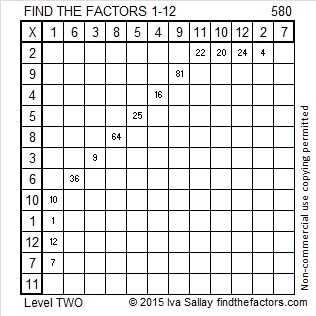# 564 and Level 6

Consecutive primes 281 and 283 add up to 564.

564 is made from three consecutive numbers so it can be evenly divided by 3. If the middle number is divisible by 3, then a number made from three consecutive numbers will also be divisible by 9. Is 564 divisible by 9? Why or why not?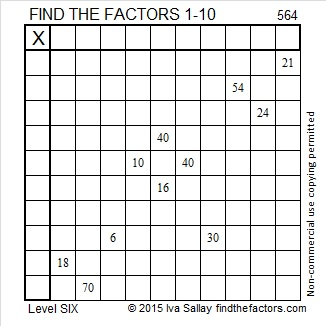Print the puzzles or type the solution on this excel file: 10 Factors 2015-07-20

—————————————————————————————————

• 564 is a composite number.
• Prime factorization: 564 = 2 x 2 x 3 x 47, which can be written 564 = (2^2) x 3 x 47
• The exponents in the prime factorization are 2, 1, and 1. Adding one to each and multiplying we get (2 + 1)(1 + 1)(1 + 1) = 3 x 2 x 2 = 12. Therefore 564 has exactly 12 factors.
• Factors of 564: 1, 2, 3, 4, 6, 12, 47, 94, 141, 188, 282, 564
• Factor pairs: 564 = 1 x 564, 2 x 282, 3 x 188, 4 x 141, 6 x 94, or 12 x 47
• Taking the factor pair with the largest square number factor, we get √564 = (√4)(√141) = 2√141 ≈ 23.74868—————————————————————————————————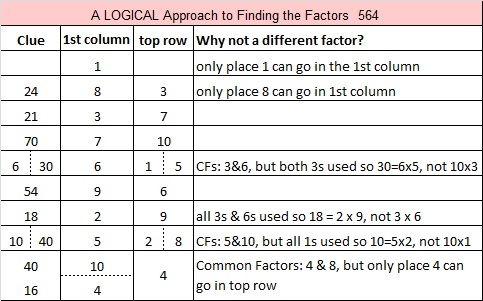# 556 and Level 6

556 is the sum of all the prime numbers from 131 to 149. One of those consecutive primes is also a prime factor of 556.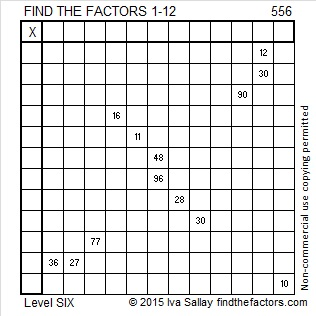Print the puzzles or type the solution on this excel file: 12 Factors 2015-07-13

—————————————————————————————————

• 556 is a composite number.
• Prime factorization: 556 = 2 x 2 x 139, which can be written 556 = (2^2) x 139
• The exponents in the prime factorization are 2 and 1. Adding one to each and multiplying we get (2 + 1)(1 + 1) = 3 x 2  = 6. Therefore 556 has exactly 6 factors.
• Factors of 556: 1, 2, 4, 139, 278, 556
• Factor pairs: 556 = 1 x 556, 2 x 278, or 4 x 139
• Taking the factor pair with the largest square number factor, we get √556 = (√4)(√139) = 2√139 ≈ 23.57965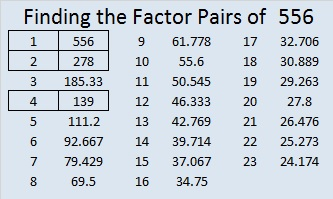—————————————————————————————————# Simplifying √539 and Level 3

If you add up all the prime numbers from 29 to 71, the sum will be 539.

Specifically, 29 + 31 + 37 + 41 + 43 + 47 + 53 + 59 + 61 + 67 + 71 = 539.

How to reduce √539: Since 539 cannot be evenly divided by 100, 4, 9, or 25, look for the smallest prime number that will divide into 539. You will soon note that 539 ÷ 7 = 77. Divide 77 by 7 again to get 11. I like to make a little cake that looks like this:Then I take the square root of everything on the outside of the cake: √539 = (√7)(√7)(√11) = 7√11Print the puzzles or type the solution on this excel file: 12 Factors 2015-06-29

—————————————————————————————————

• 539 is a composite number.
• Prime factorization: 539 = 7 x 7 x 11, which can be written 539 = (7^2) x 11
• The exponents in the prime factorization are 2 and 1. Adding one to each and multiplying we get (2 + 1)(1 + 1) = 3 x 2  = 6. Therefore 539 has exactly 6 factors.
• Factors of 539: 1, 7, 11, 49, 77, 539
• Factor pairs: 539 = 1 x 539, 7 x 77, or 11 x 49
• Taking the factor pair with the largest square number factor, we get √539 = (√49)(√11) = 7√11 ≈ 23.21637353—————————————————————————————————

A Logical Approach to solve a FIND THE FACTORS puzzle: Find the column or row with two clues and find their common factor. Write the corresponding factors in the factor column (1st column) and factor row (top row).  Because this is a level three puzzle, you have now written a factor at the top of the factor column. Continue to work from the top of the factor column to the bottom, finding factors and filling in the factor column and the factor row one cell at a time as you go.# 534 and Level 5

534 is made of three consecutive digits so it can be evenly divided by three.

534 is the sum of consecutive primes: 127 + 131 + 137 + 139 = 534.

534 is the hypotenuse of the Pythagorean triple 234-480-534. Can you find the greatest common factor of those three numbers?Print the puzzles or type the solution on this excel file: 10 Factors 2015-06-22

—————————————————————————————————

• 534 is a composite number.
• Prime factorization: 534 = 2 x 3 x 89
• The exponents in the prime factorization are 1, 1, and 1. Adding one to each and multiplying we get (1 + 1)(1 + 1)(1 + 1) = 2 x 2 x 2 = 8. Therefore 534 has exactly 8 factors.
• Factors of 534: 1, 2, 3, 6, 89, 178, 267, 534
• Factor pairs: 534 = 1 x 534, 2 x 267, 3 x 178, or 6 x 89
• 534 has no square factors that allow its square root to be simplified. √534 ≈ 23.108440.—————————————————————————————————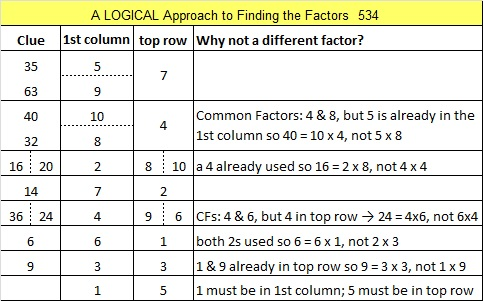# 533 and Level 4

533 is the sum of consecutive primes two different ways: 173 + 179 + 181 = 533 = 101 + 103 + 107 + 109 + 113.

533 = (23^2) + (2^2), and 533 = (22^2) + (7^2)

533 is the hypotenuse of four Pythagorean triples. Some of the triples have a greatest common factor greater than one, and the rest are primitive. Which are which?

• 92-525-533
• 117-520-533
• 205-492-533
• 308-435-533Print the puzzles or type the solution on this excel file: 10 Factors 2015-06-22

—————————————————————————————————

• 533 is a composite number.
• Prime factorization: 533 = 13 x 41
• The exponents in the prime factorization are 1 and 1. Adding one to each and multiplying we get (1 + 1)(1 + 1) = 2 x 2 = 4. Therefore 533 has exactly 4 factors.
• Factors of 533: 1, 13, 41, 533
• Factor pairs: 533 = 1 x 533 or 13 x 41
• 533 has no square factors that allow its square root to be simplified. √533 ≈ 23.08679—————————————————————————————————# 532 and Level 3

532 is the sum of consecutive prime numbers 263 and 269.

532 is also the 19th pentagonal number.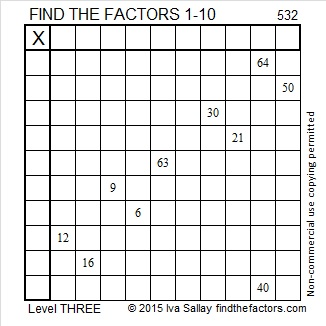Print the puzzles or type the solution on this excel file: 10 Factors 2015-06-22

—————————————————————————————————

• 532 is a composite number.
• Prime factorization: 532 = 2 x 2 x 7 x 19, which can be written 532 = (2^2) x 7 x 19
• The exponents in the prime factorization are 1, 2, and 1. Adding one to each and multiplying we get (2 + 1)(1 + 1)(1 + 1) = 3 x 2 x 2 = 12. Therefore 532 has exactly 12 factors.
• Factors of 532: 1, 2, 4, 7, 14, 19, 28, 38, 76, 133, 266, 532
• Factor pairs: 532 = 1 x 532, 2 x 266, 4 x 133, 7 x 76, 14 x 38, or 19 x 28
• Taking the factor pair with the largest square number factor, we get √532 = (√4)(√133) = 2√133 ≈ 23.065125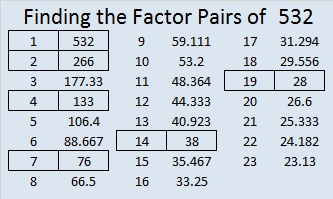—————————————————————————————————

A Logical Approach to solve a FIND THE FACTORS puzzle: Find the column or row with two clues and find their common factor. Write the corresponding factors in the factor column (1st column) and factor row (top row).  Because this is a level three puzzle, you have now written a factor at the top of the factor column. Continue to work from the top of the factor column to the bottom, finding factors and filling in the factor column and the factor row one cell at a time as you go.# 531 and Level 2

5 + 3 + 1 = 9 so 531 is divisible by 3 and by 9. Because it can be evenly divided by 9, it’s square root can be reduced.

531 ÷ 9 = 59, a prime number which obviously has no square factors. Thus √531 = (√9)(√59) = 3√59 in its most reduced form.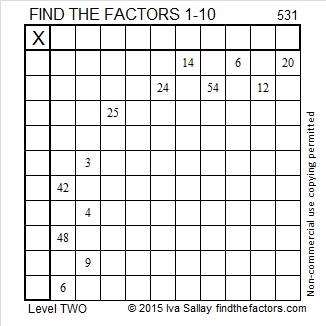Print the puzzles or type the solution on this excel file: 10 Factors 2015-06-22

—————————————————————————————————

• 531 is a composite number.
• Prime factorization: 531 = 3 x 3 x 59, which can be written 531 = (3^2) x 59
• The exponents in the prime factorization are 2 and 1. Adding one to each and multiplying we get (2 + 1)(1 + 1) = 3 x 2  = 6. Therefore 531 has exactly 6 factors.
• Factors of 531: 1, 3, 9, 59, 177, 531
• Factor pairs: 531 = 1 x 531, 3 x 177, or 9 x 59
• Taking the factor pair with the largest square number factor, we get √531 = (√9)(√59) = 3√59 ≈ 23.04343724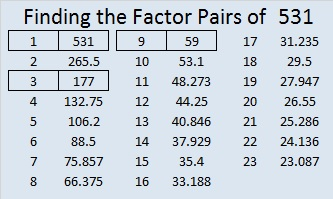—————————————————————————————————